# Contracts with Interdependent Preferences

Mar. 22, 2023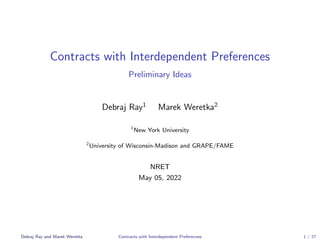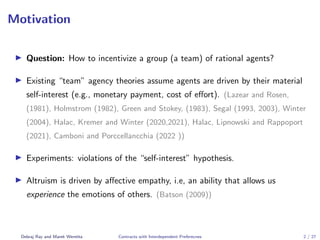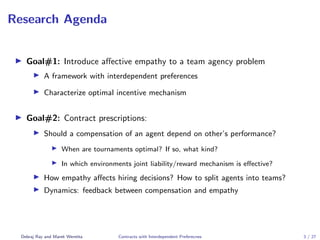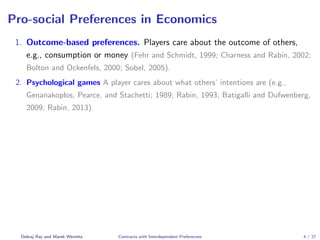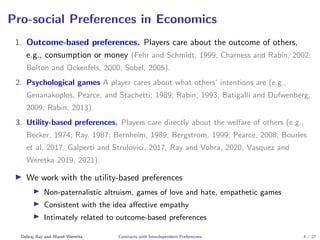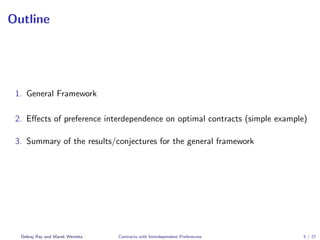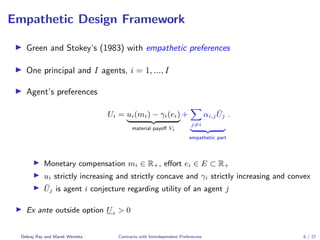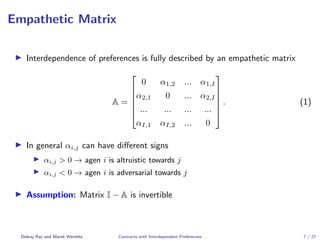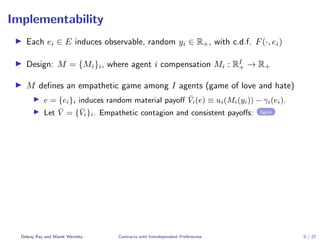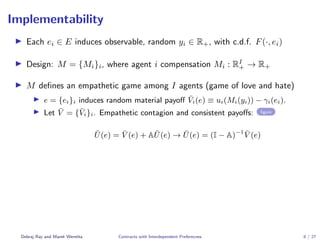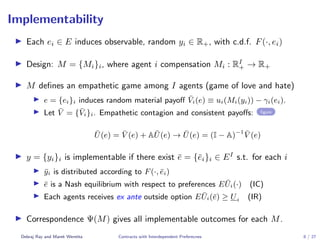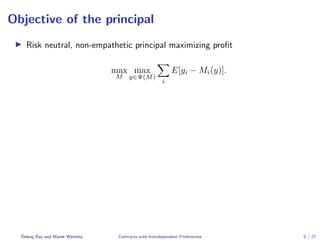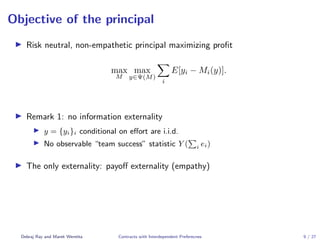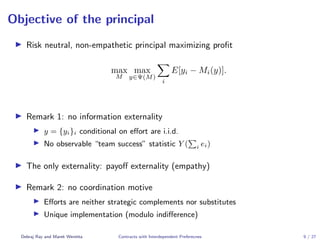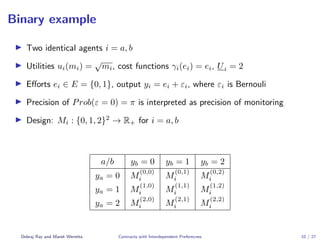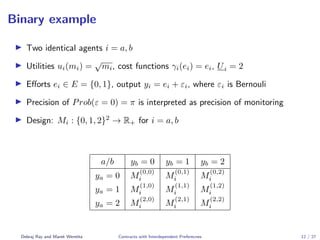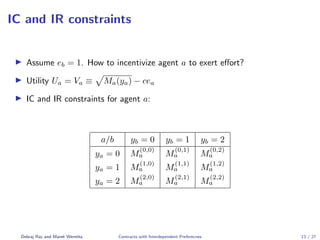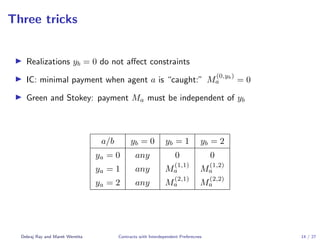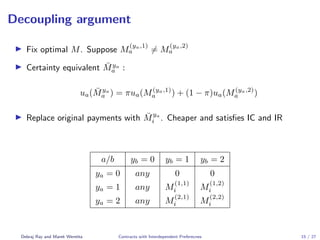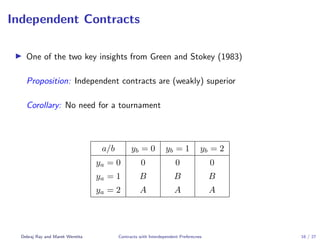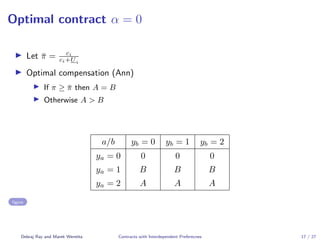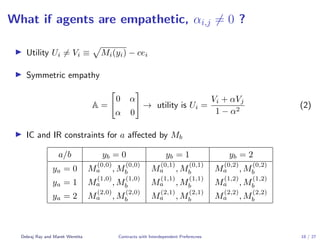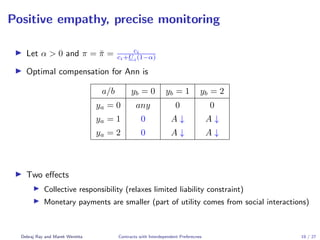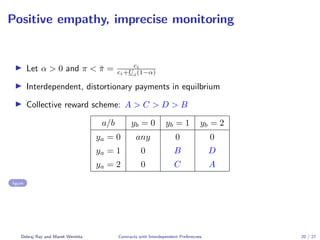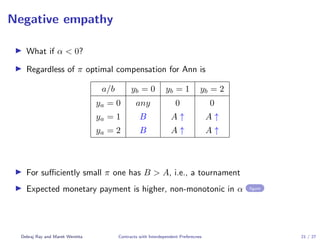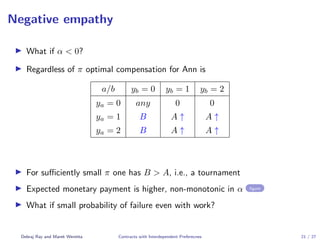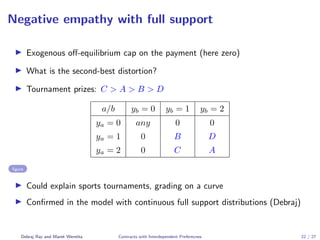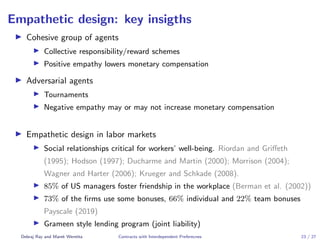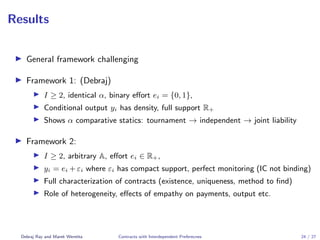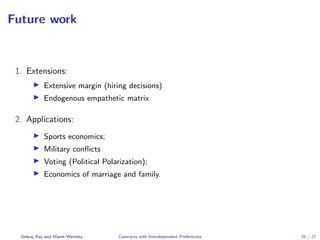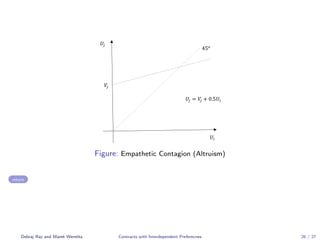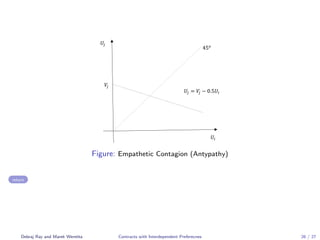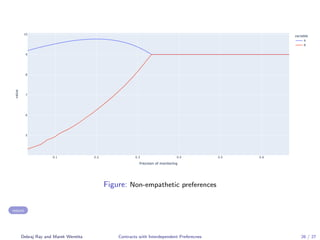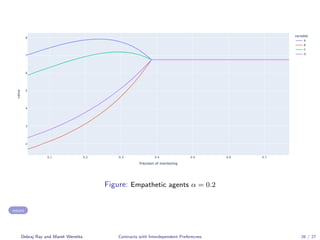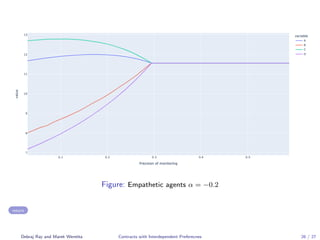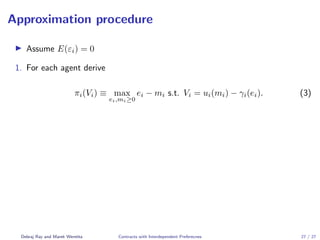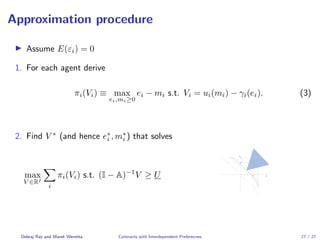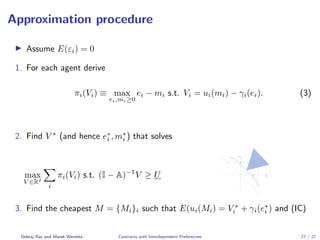1 of 44

### Contracts with Interdependent Preferences

1. Contracts with Interdependent Preferences Preliminary Ideas Debraj Ray1 Marek Weretka2 1 New York University 2 University of Wisconsin-Madison and GRAPE/FAME NRET May 05, 2022 Debraj Ray and Marek Weretka Contracts with Interdependent Preferecnes 1 / 27
2. Motivation I Question: How to incentivize a group (a team) of rational agents? I Existing “team” agency theories assume agents are driven by their material self-interest (e.g., monetary payment, cost of effort). (Lazear and Rosen, (1981), Holmstrom (1982), Green and Stokey, (1983), Segal (1993, 2003), Winter (2004), Halac, Kremer and Winter (2020,2021), Halac, Lipnowski and Rappoport (2021), Camboni and Porccellancchia (2022 )) I Experiments: violations of the “self-interest” hypothesis. I Altruism is driven by affective empathy, i.e, an ability that allows us experience the emotions of others. (Batson (2009)) Debraj Ray and Marek Weretka Contracts with Interdependent Preferecnes 2 / 27
3. Research Agenda I Goal#1: Introduce affective empathy to a team agency problem I A framework with interdependent preferences I Characterize optimal incentive mechanism I Goal#2: Contract prescriptions: I Should a compensation of an agent depend on other’s performance? I When are tournaments optimal? If so, what kind? I In which environments joint liability/reward mechanism is effective? I How empathy affects hiring decisions? How to split agents into teams? I Dynamics: feedback between compensation and empathy Debraj Ray and Marek Weretka Contracts with Interdependent Preferecnes 3 / 27
4. Pro-social Preferences in Economics 1. Outcome-based preferences. Players care about the outcome of others, e.g., consumption or money (Fehr and Schmidt, 1999; Charness and Rabin, 2002; Bolton and Ockenfels, 2000; Sobel, 2005). 2. Psychological games A player cares about what others’ intentions are (e.g., Genanakoplos, Pearce, and Stachetti; 1989; Rabin, 1993; Batigalli and Dufwenberg, 2009; Rabin, 2013). Debraj Ray and Marek Weretka Contracts with Interdependent Preferecnes 4 / 27
5. Pro-social Preferences in Economics 1. Outcome-based preferences. Players care about the outcome of others, e.g., consumption or money (Fehr and Schmidt, 1999; Charness and Rabin, 2002; Bolton and Ockenfels, 2000; Sobel, 2005). 2. Psychological games A player cares about what others’ intentions are (e.g., Genanakoplos, Pearce, and Stachetti; 1989; Rabin, 1993; Batigalli and Dufwenberg, 2009; Rabin, 2013). 3. Utility-based preferences. Players care directly about the welfare of others (e.g., Becker, 1974; Ray, 1987; Bernheim, 1989; Bergstrom, 1999; Pearce, 2008; Bourles et al, 2017, Galperti and Strulovici, 2017, Ray and Vohra, 2020, Vasquez and Weretka 2019, 2021). I We work with the utility-based preferences I Non-paternalistic altruism, games of love and hate, empathetic games I Consistent with the idea affective empathy I Intimately related to outcome-based preferences Debraj Ray and Marek Weretka Contracts with Interdependent Preferecnes 4 / 27
6. Outline 1. General Framework 2. Effects of preference interdependence on optimal contracts (simple example) 3. Summary of the results/conjectures for the general framework Debraj Ray and Marek Weretka Contracts with Interdependent Preferecnes 5 / 27
7. Empathetic Design Framework I Green and Stokey’s (1983) with empathetic preferences I One principal and I agents, i = 1, ..., I I Agent’s preferences Ui = ui(mi) − γi(ei) | {z } material payoff Vi + X j6=i αi,jŪj | {z } empathetic part . I Monetary compensation mi ∈ R+, effort ei ∈ E ⊂ R+ I ui strictly increasing and strictly concave and γi strictly increasing and convex I Ūj is agent i conjecture regarding utility of an agent j I Ex ante outside option Ui > 0 Debraj Ray and Marek Weretka Contracts with Interdependent Preferecnes 6 / 27
8. Empathetic Matrix I Interdependence of preferences is fully described by an empathetic matrix A =       0 α1,2 ... α1,I α2,1 0 ... α2,I ... ... ... ... αI,1 αI,2 ... 0       . (1) I In general αi,j can have different signs I αi,j > 0 → agen i is altruistic towards j I αi,j < 0 → agen i is adversarial towards j I Assumption: Matrix I − A is invertible Debraj Ray and Marek Weretka Contracts with Interdependent Preferecnes 7 / 27
9. Implementability I Each ei ∈ E induces observable, random yi ∈ R+, with c.d.f. F(·, ei) I Design: M = {Mi}i, where agent i compensation Mi : RI + → R+ I M defines an empathetic game among I agents (game of love and hate) I e = {ei}i induces random material payoff V̄i(e) ≡ ui(Mi(yi)) − γi(ei). I Let V̄ = {V̄i}i. Empathetic contagion and consistent payoffs: figure Debraj Ray and Marek Weretka Contracts with Interdependent Preferecnes 8 / 27
10. Implementability I Each ei ∈ E induces observable, random yi ∈ R+, with c.d.f. F(·, ei) I Design: M = {Mi}i, where agent i compensation Mi : RI + → R+ I M defines an empathetic game among I agents (game of love and hate) I e = {ei}i induces random material payoff V̄i(e) ≡ ui(Mi(yi)) − γi(ei). I Let V̄ = {V̄i}i. Empathetic contagion and consistent payoffs: figure Ū(e) = V̄ (e) + AŪ(e) → Ū(e) = (I − A)−1 V̄ (e) Debraj Ray and Marek Weretka Contracts with Interdependent Preferecnes 8 / 27
11. Implementability I Each ei ∈ E induces observable, random yi ∈ R+, with c.d.f. F(·, ei) I Design: M = {Mi}i, where agent i compensation Mi : RI + → R+ I M defines an empathetic game among I agents (game of love and hate) I e = {ei}i induces random material payoff V̄i(e) ≡ ui(Mi(yi)) − γi(ei). I Let V̄ = {V̄i}i. Empathetic contagion and consistent payoffs: figure Ū(e) = V̄ (e) + AŪ(e) → Ū(e) = (I − A)−1 V̄ (e) I y = {yi}i is implementable if there exist ē = {ēi}i ∈ EI s.t. for each i I ȳi is distributed according to F(·, ēi) I ē is a Nash equilibrium with respect to preferences EŪi(·) (IC) I Each agents receives ex ante outside option EŪi(ē) ≥ Ui (IR) I Correspondence Ψ(M) gives all implementable outcomes for each M. Debraj Ray and Marek Weretka Contracts with Interdependent Preferecnes 8 / 27
12. Objective of the principal I Risk neutral, non-empathetic principal maximizing profit max M max y∈Ψ(M) X i E[yi − Mi(y)]. Debraj Ray and Marek Weretka Contracts with Interdependent Preferecnes 9 / 27
13. Objective of the principal I Risk neutral, non-empathetic principal maximizing profit max M max y∈Ψ(M) X i E[yi − Mi(y)]. I Remark 1: no information externality I y = {yi}i conditional on effort are i.i.d. I No observable “team success” statistic Y ( P i ei) I The only externality: payoff externality (empathy) Debraj Ray and Marek Weretka Contracts with Interdependent Preferecnes 9 / 27
14. Objective of the principal I Risk neutral, non-empathetic principal maximizing profit max M max y∈Ψ(M) X i E[yi − Mi(y)]. I Remark 1: no information externality I y = {yi}i conditional on effort are i.i.d. I No observable “team success” statistic Y ( P i ei) I The only externality: payoff externality (empathy) I Remark 2: no coordination motive I Efforts are neither strategic complements nor substitutes I Unique implementation (modulo indifference) Debraj Ray and Marek Weretka Contracts with Interdependent Preferecnes 9 / 27
15. Binary example I Two identical agents i = a, b I Utilities ui(mi) = √ mi, cost functions γi(ei) = ei, Ui = 2 I Efforts ei ∈ E = {0, 1}, output yi = ei + εi, where εi is Bernouli I Precision of Prob(ε = 0) = π is interpreted as precision of monitoring I Design: Mi : {0, 1, 2}2 → R+ for i = a, b a/b yb = 0 yb = 1 yb = 2 ya = 0 M (0,0) i M (0,1) i M (0,2) i ya = 1 M (1,0) i M (1,1) i M (1,2) i ya = 2 M (2,0) i M (2,1) i M (2,2) i Debraj Ray and Marek Weretka Contracts with Interdependent Preferecnes 10 / 27
16. Binary example I Two identical agents i = a, b I Utilities ui(mi) = √ mi, cost functions γi(ei) = ei, Ui = 2 I Efforts ei ∈ E = {0, 1}, output yi = ei + εi, where εi is Bernouli I Precision of Prob(ε = 0) = π is interpreted as precision of monitoring I Design: Mi : {0, 1, 2}2 → R+ for i = a, b a/b yb = 0 yb = 1 yb = 2 ya = 0 M (0,0) i M (0,1) i M (0,2) i ya = 1 M (1,0) i M (1,1) i M (1,2) i ya = 2 M (2,0) i M (2,1) i M (2,2) i Debraj Ray and Marek Weretka Contracts with Interdependent Preferecnes 11 / 27
17. Binary example I Two identical agents i = a, b I Utilities ui(mi) = √ mi, cost functions γi(ei) = ei, Ui = 2 I Efforts ei ∈ E = {0, 1}, output yi = ei + εi, where εi is Bernouli I Precision of Prob(ε = 0) = π is interpreted as precision of monitoring I Design: Mi : {0, 1, 2}2 → R+ for i = a, b a/b yb = 0 yb = 1 yb = 2 ya = 0 M (0,0) i M (0,1) i M (0,2) i ya = 1 M (1,0) i M (1,1) i M (1,2) i ya = 2 M (2,0) i M (2,1) i M (2,2) i Debraj Ray and Marek Weretka Contracts with Interdependent Preferecnes 12 / 27
18. IC and IR constraints I Assume eb = 1. How to incentivize agent a to exert effort? I Utility Ua = Va ≡ p Ma(ya) − cea I IC and IR constraints for agent a: a/b yb = 0 yb = 1 yb = 2 ya = 0 M (0,0) a M (0,1) a M (0,2) a ya = 1 M (1,0) a M (1,1) a M (1,2) a ya = 2 M (2,0) a M (2,1) a M (2,2) a Debraj Ray and Marek Weretka Contracts with Interdependent Preferecnes 13 / 27
19. Three tricks I Realizations yb = 0 do not affect constraints I IC: minimal payment when agent a is “caught:” M (0,yb) a = 0 I Green and Stokey: payment Ma must be independent of yb a/b yb = 0 yb = 1 yb = 2 ya = 0 any 0 0 ya = 1 any M (1,1) a M (1,2) a ya = 2 any M (2,1) a M (2,2) a Debraj Ray and Marek Weretka Contracts with Interdependent Preferecnes 14 / 27
20. Decoupling argument I Fix optimal M. Suppose M (ya,1) a 6= M (ya,2) a I Certainty equivalent M̄ya a : ua(M̄ya a ) = πua(M(ya,1) a ) + (1 − π)ua(M(ya,2) a ) I Replace original payments with M̄ya i . Cheaper and satisfies IC and IR a/b yb = 0 yb = 1 yb = 2 ya = 0 any 0 0 ya = 1 any M (1,1) i M (1,2) i ya = 2 any M (2,1) i M (2,2) i Debraj Ray and Marek Weretka Contracts with Interdependent Preferecnes 15 / 27
21. Independent Contracts I One of the two key insights from Green and Stokey (1983) Proposition: Independent contracts are (weakly) superior Corollary: No need for a tournament a/b yb = 0 yb = 1 yb = 2 ya = 0 0 0 0 ya = 1 B B B ya = 2 A A A Debraj Ray and Marek Weretka Contracts with Interdependent Preferecnes 16 / 27
22. Optimal contract α = 0 I Let π̄ = ci ci+Ui I Optimal compensation (Ann) I If π ≥ π̄ then A = B I Otherwise A > B a/b yb = 0 yb = 1 yb = 2 ya = 0 0 0 0 ya = 1 B B B ya = 2 A A A figure Debraj Ray and Marek Weretka Contracts with Interdependent Preferecnes 17 / 27
23. What if agents are empathetic, αi,j 6= 0 ? I Utility Ui 6= Vi ≡ p Mi(yi) − cei I Symmetric empathy A = " 0 α α 0 # → utility is Ui = Vi + αVj 1 − α2 (2) I IC and IR constraints for a affected by Mb a/b yb = 0 yb = 1 yb = 2 ya = 0 M (0,0) a , M (0,0) b M (0,1) a , M (0,1) b M (0,2) a , M (0,2) b ya = 1 M (1,0) a , M (1,0) b M (1,1) a , M (1,1) b M (1,2) a , M (1,2) b ya = 2 M (2,0) a , M (2,0) b M (2,1) a , M (2,1) b M (2,2) a , M (2,2) b Debraj Ray and Marek Weretka Contracts with Interdependent Preferecnes 18 / 27
24. Positive empathy, precise monitoring I Let α > 0 and π = π̄ = ci ci+Ui(1−α) I Optimal compensation for Ann is a/b yb = 0 yb = 1 yb = 2 ya = 0 any 0 0 ya = 1 0 A ↓ A ↓ ya = 2 0 A ↓ A ↓ I Two effects I Collective responsibility (relaxes limited liability constraint) I Monetary payments are smaller (part of utility comes from social interactions) Debraj Ray and Marek Weretka Contracts with Interdependent Preferecnes 19 / 27
25. Positive empathy, imprecise monitoring I Let α > 0 and π < π̄ = ci ci+Ui(1−α) I Interdependent, distortionary payments in equilbrium I Collective reward scheme: A > C > D > B a/b yb = 0 yb = 1 yb = 2 ya = 0 any 0 0 ya = 1 0 B D ya = 2 0 C A figure Debraj Ray and Marek Weretka Contracts with Interdependent Preferecnes 20 / 27
26. Negative empathy I What if α < 0? I Regardless of π optimal compensation for Ann is a/b yb = 0 yb = 1 yb = 2 ya = 0 any 0 0 ya = 1 B A ↑ A ↑ ya = 2 B A ↑ A ↑ I For sufficiently small π one has B > A, i.e., a tournament I Expected monetary payment is higher, non-monotonic in α figure Debraj Ray and Marek Weretka Contracts with Interdependent Preferecnes 21 / 27
27. Negative empathy I What if α < 0? I Regardless of π optimal compensation for Ann is a/b yb = 0 yb = 1 yb = 2 ya = 0 any 0 0 ya = 1 B A ↑ A ↑ ya = 2 B A ↑ A ↑ I For sufficiently small π one has B > A, i.e., a tournament I Expected monetary payment is higher, non-monotonic in α figure I What if small probability of failure even with work? Debraj Ray and Marek Weretka Contracts with Interdependent Preferecnes 21 / 27
28. Negative empathy with full support I Exogenous off-equilibrium cap on the payment (here zero) I What is the second-best distortion? I Tournament prizes: C > A > B > D a/b yb = 0 yb = 1 yb = 2 ya = 0 any 0 0 ya = 1 0 B D ya = 2 0 C A figure I Could explain sports tournaments, grading on a curve I Confirmed in the model with continuous full support distributions (Debraj) Debraj Ray and Marek Weretka Contracts with Interdependent Preferecnes 22 / 27
29. Empathetic design: key insigths I Cohesive group of agents I Collective responsibility/reward schemes I Positive empathy lowers monetary compensation I Adversarial agents I Tournaments I Negative empathy may or may not increase monetary compensation I Empathetic design in labor markets I Social relationships critical for workers’ well-being. Riordan and Griffeth (1995); Hodson (1997); Ducharme and Martin (2000); Morrison (2004); Wagner and Harter (2006); Krueger and Schkade (2008). I 85% of US managers foster friendship in the workplace (Berman et al. (2002)) I 73% of the firms use some bonuses, 66% individual and 22% team bonuses Payscale (2019) I Grameen style lending program (joint liability) Debraj Ray and Marek Weretka Contracts with Interdependent Preferecnes 23 / 27
30. Results I General framework challenging I Framework 1: (Debraj) I I ≥ 2, identical α, binary effort ei = {0, 1}, I Conditional output yi has density, full support R+ I Shows α comparative statics: tournament → independent → joint liability I Framework 2: I I ≥ 2, arbitrary A, effort ei ∈ R+, I yi = ei + εi where εi has compact support, perfect monitoring (IC not binding) I Full characterization of contracts (existence, uniqueness, method to find) I Role of heterogeneity, effects of empathy on payments, output etc. Debraj Ray and Marek Weretka Contracts with Interdependent Preferecnes 24 / 27
31. Future work 1. Extensions: I Extensive margin (hiring decisions) I Endogenous empathetic matrix 2. Applications: I Sports economics; I Military conflicts I Voting (Political Polarization); I Economics of marriage and family. Debraj Ray and Marek Weretka Contracts with Interdependent Preferecnes 25 / 27
32. Thank you! Debraj Ray and Marek Weretka Contracts with Interdependent Preferecnes 26 / 27
33. 45! # " \$" \$# \$" = # " + 0.5\$# Figure: Empathetic Contagion (Altruism) return Debraj Ray and Marek Weretka Contracts with Interdependent Preferecnes 26 / 27
34. 45! # " \$" \$# \$" = # " + 0.5\$# Figure: Empathetic Contagion (Altruism) return Debraj Ray and Marek Weretka Contracts with Interdependent Preferecnes 26 / 27
35. 45! # " \$" \$# \$" = # " + 0.5\$# Figure: Empathetic Contagion (Altruism) return Debraj Ray and Marek Weretka Contracts with Interdependent Preferecnes 26 / 27
36. 45! # " \$" \$# \$" = # " + 0.5\$# Figure: Empathetic Contagion (Altruism) return Debraj Ray and Marek Weretka Contracts with Interdependent Preferecnes 26 / 27
37. 45! # " \$" \$# \$" = # " − 0.5\$# Figure: Empathetic Contagion (Antypathy) return Debraj Ray and Marek Weretka Contracts with Interdependent Preferecnes 26 / 27
38. Figure: Non-empathetic preferences return Debraj Ray and Marek Weretka Contracts with Interdependent Preferecnes 26 / 27
39. Figure: Empathetic agents α = 0.2 return Debraj Ray and Marek Weretka Contracts with Interdependent Preferecnes 26 / 27
40. Figure: Empathetic agents α = −0.2 return Debraj Ray and Marek Weretka Contracts with Interdependent Preferecnes 26 / 27
41. Figure: Expected payment return Debraj Ray and Marek Weretka Contracts with Interdependent Preferecnes 26 / 27
42. Approximation procedure I Assume E(εi) = 0 1. For each agent derive πi(Vi) ≡ max ei,mi≥0 ei − mi s.t. Vi = ui(mi) − γi(ei). (3) Debraj Ray and Marek Weretka Contracts with Interdependent Preferecnes 27 / 27
43. Approximation procedure I Assume E(εi) = 0 1. For each agent derive πi(Vi) ≡ max ei,mi≥0 ei − mi s.t. Vi = ui(mi) − γi(ei). (3) 2. Find V ∗ (and hence e∗ i , m∗ i ) that solves max V ∈RI X i πi(Vi) s.t. (I − A)−1 V ≥ U 𝑣2 4 𝑣1 4 Debraj Ray and Marek Weretka Contracts with Interdependent Preferecnes 27 / 27
44. Approximation procedure I Assume E(εi) = 0 1. For each agent derive πi(Vi) ≡ max ei,mi≥0 ei − mi s.t. Vi = ui(mi) − γi(ei). (3) 2. Find V ∗ (and hence e∗ i , m∗ i ) that solves max V ∈RI X i πi(Vi) s.t. (I − A)−1 V ≥ U 𝑣2 4 𝑣1 4 3. Find the cheapest M = {Mi}i such that E(ui(Mi) = V ∗ i + γi(e∗ i ) and (IC) Debraj Ray and Marek Weretka Contracts with Interdependent Preferecnes 27 / 27